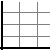## Default Prediction for Companies

Certain fundamental analysis models can be to predict the probability that a company will default on its debt or go bankrupt in a given period of time. This resource will eventually use the following three models to predict when companies are close to the default point:

• Z - Score Model
• Vaseick Kealhofer Model(EDF Credit measure)
• Black - Scholes Model

## The Z-Score Model

MDA(Multiple Discriminant Analysis) is a statistical technique used to classify an observation into one of several a priori groupings dependent upon the observation's individual characteristics. It is used primarily to classify and make predictions in problems where the dependent variable appears in qualitative form, for example, bankrupt or non bankrupt. Therefore the first step is to establish group classifications.
After the groups are established, data are collected for the objects in the groups. MDA in its most simple form attempts to derive a linear combination of these characteristics which "best" discriminates between the groups.If a particular object,for instance, a corporation, has characteristics(financial ratios) which can be quantified for all the companies in the analysis the MDA determines a set of discriminant coefficients. When these coefficients are applied to the actual ratios, a basis for classification into one of the mutually exclusive grouping exists.
The discriminant function of the form Z= V1X1 + V2X2 +...+VnXn transforms the individual variable values to a single discriminant score , or Z value, which is then used to classify the object where V1,V2,...Vn = discriminant coefficients X1,X2....Xn = independent variables

The Z-Score model is a linear analysis in that five measures are objectively weighted and summed up to arrive at an overall score that then becomes the basis for classification of firms into one of the a priori groupings(distressed and non distressed).

After initial groups are defined and firms selected, balance sheet and income statement data are collected. A list of 22 potentially helpful ratios was compiled for evaluation and the 5 of them were finally chosen for doing the best overall job together in the prediction.The following procedures are utilized: (1)observation of the statistical significance of various alternative functions,including determination of the relative contributions of each independent variable.(2)evaluation of intercorrelations among the relevant variables(3)observation of the predictive accuracy of the various profiles and (4) subjective judgment The ratios are classified into five standard categories, including liquidity, profitability,leverage,solvency,activity.
The final discriminant function is as follows:

Z= 1.2X1 + 1.4X2 + 3.3X3 + 0.6X4 + 0.999X5

where

X1 = working capital/total Assets
X2 = retained earnings/total Assets
X3 = earnings before interests and taxes/total Assets
X4 = market value of equity/book value of total liabilities
X5 = sales/total Assets
Z = overall index

X1(Working Capital/Total Assets)
The working capital/total assets ratio is a measure of the net liquid assets of the firm relative to the total capitalization. Working capital is defined as the difference between current assets and current liabilities. Ordinarily a firm experiencing consistent operating losses will have shrinking current assets in relation to total assets.

X2(Retained Earnings/Total Assets)
Retained earnings is the account which reports the total amount of reinvested earnings and/or losses of a firm over its entire life. The account is also referred to as earned surplus. The RE/TA ratio measures the leverage of a firm. Those firms with high RE, relative to TA, have financed their assets through retention of profits and have not utilized as much debt.

X3(Earnings before Interest and Taxes/Total Assets)
This ratio is a measure of the true productivity of the firm's assets,independent of any tax or leverage factors. Since a firm's ultimate existence is based on the earning power of its assets, this ratio appears to be particularly appropriate for studies dealing with corporate failure.

X4(Market Value of Equity/Book Value of Liabilities)
Equity is measured by the combined value of all shares of stock,preferred and common,while liabilities include both current and long term.The measure shows how much the firm's assets can decline in value before the liabilities exceed the assets and the firm becomes insolvent.

X5(Sales/Total Assets)
The capital-turnover ratio is a standard financial ratio illustrating the sales generating ability of the firm's assets. It is one measure of management's capacity in dealing with competitive conditions.

The resulting Z-Score puts a company in one of three categories. Companies with a Z-Score above 3.0 are considered healthy. A Z-Score less than 1.8 indicates a high probability for bankruptcy in the next 1-2 years. Scores of 1.8-3.0 are considered within the "gray area".

There are two revisions of the Z-Score that are designed to apply to private companies. For public companies the original Z-score should be preferred.

## The Revised Z-Score model for Private manufacturing companies

A complete re estimation of the model occurs by substituting the book values of equity for the Market value in X4. All the coefficients change and the classification criterion and related cut-off scores also change. The revised Z score is as follows:

Z' = 0.717X1 + 0.847X2 + 3.107X3 +0.420X4 +0.998X5

This score is recommended for private manufacturing companies.

## A Further Revision for Private non-manufacturing companies

The next modification of the Z-score analyzed the characteristics and accuracy of a model without X5 - sales/total assets.We do this to minimize the potential industry effect which is more likely to take place when such an industry sensitive variable as asset turnover is included.The new Z score is as follows:

Z'' = 6.56X1 + 3.26X2 +6.72X3 +1.05X4

The Z'' score is recommended for private non-manufacturing companies.

## Note

This part of the TextBiz analysis is inspired by the CSE691 Default Prediction project by M. Verma which can be found [here].